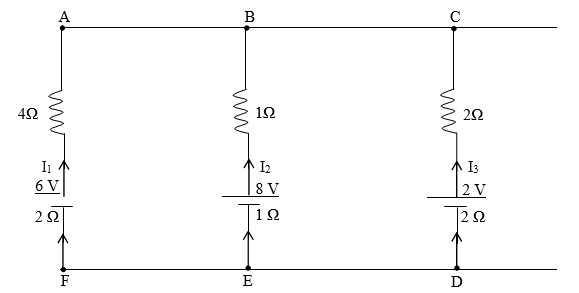# ISC Class 12 Physics Important Questions

Class 12 physics is one such subject that requires a lot of practice and dedication to get through. With a lot of numerical included, the subject needs a great amount of problem-solving and analytical skills. The ISC Class 12 physics syllabus is very vast and hence memorising each of them is often very difficult. To ease the effort of students preparing for the exams, the specialists at BYJU’S have come up with the ISC Class 12 important questions. The list of questions provided in it covers almost all the important topics and concepts that are most likely to appear for the ISC class 12 exam.

### The Important Questions of ISC Class 12 Physics are given below:

1. Show how you will obtain an AND gate using only NOR gates. Draw the truth table for this arrangement of gates.
2. For a common emitter transistor amplifier, the audio signal voltage across the collector resistance (rc ) of 2 kΩ is 2 V. If the current amplification factor (β) of the transistor is 100, calculate the input signal voltage (VBE) and base current (IB) for base resistance of 1 kΩ.
3. For a transistor in a common emitter mode, draw a labelled graph to show:
1. Input characteristic curve.
2. Output characteristic curve.
3. Transfer characteristic curve.
4. Draw a neat and labelled diagram of an experimental setup of Young’s double slit experiment to study the interference of light and show that:β=λD/d

Where the terms have their usual meaning. Show intensity variation in the interference.

5. Draw a labelled ray diagram of an image formed by a compound microscope with a final image formed at the least distance of distinct vision (D). Derive an expression for its magnifying power (in terms of Vo, Uo, fe and D).
6. An 8 H inductor, a 2 μF capacitor and a 100 resistor are connected in series to an A.C. supply of 220 V and 50 Hz. Calculate:
1. The impedance of the circuit.
2. Current flowing through the circuit.
3. The phase difference between the current and the supply voltage.
4. Average power consumed by the circuit.
7. With reference to a semiconductor diode, define the terms ‘depletion region’ and ‘potential barrier’. How will the width of depletion region change during reverse biasing?
8. When a ray of ordinary light is incident on the surface of separation of two media at the polarizing angle, show with the help of a labelled diagram that reflected ray and the refracted ray are mutually perpendicular to each other.
9. Derive R = 2f for a spherical mirror, where the symbols have their usual meaning.
10. Using Kirchhoff’s laws of electrical networks, calculate the currents I1, I2 and I3 in the circuit shown below11. A parallel plate capacitor is charged by a battery; which is then disconnected. A dielectric slab is now introduced between the two plates to occupy the space completely. State the effect on the following:
1. The capacitance of the capacitor.
2. The potential difference between the plates.
3. The energy stored in the capacitor.
12. Obtain an expression for electric potential ‘V’ due to a point charge ‘Q’ at a distance r.
13. Using Gauss’ theorem, obtain an expression for the intensity of electric field ‘E’ at a point, which is at a distance „r‟ (r > R) from the centre „C‟ of a thin spherical shell (of radius R) carrying charge „Q‟.
14. Name the essential components of a communication system. Draw its block diagram.
15. What is meant by ‘binding energy per nucleon’ of a nucleus? State its physical significance.
16. Draw energy level diagram for Hydrogen atom showing at least four lowest energy levels. Show the transitions responsible for the emission of the Balmer series.
17. Plot a labelled graph of the maximum kinetic energy of photoelectrons versus frequency of incident radiation. State how you will obtain the value of Planck‟s constant „h‟ from the graph.
18. What is meant by dispersive power? Write an expression of dispersive power in terms of refractive indices.
19. A thin convex lens of focal length 20 cm is kept in contact with a thin concave lens of focal length 15 cm. Find the focal length and the nature of the combination.
1. What is the displacement current?
2. Which electromagnetic radiation is used to study the crystal structure?